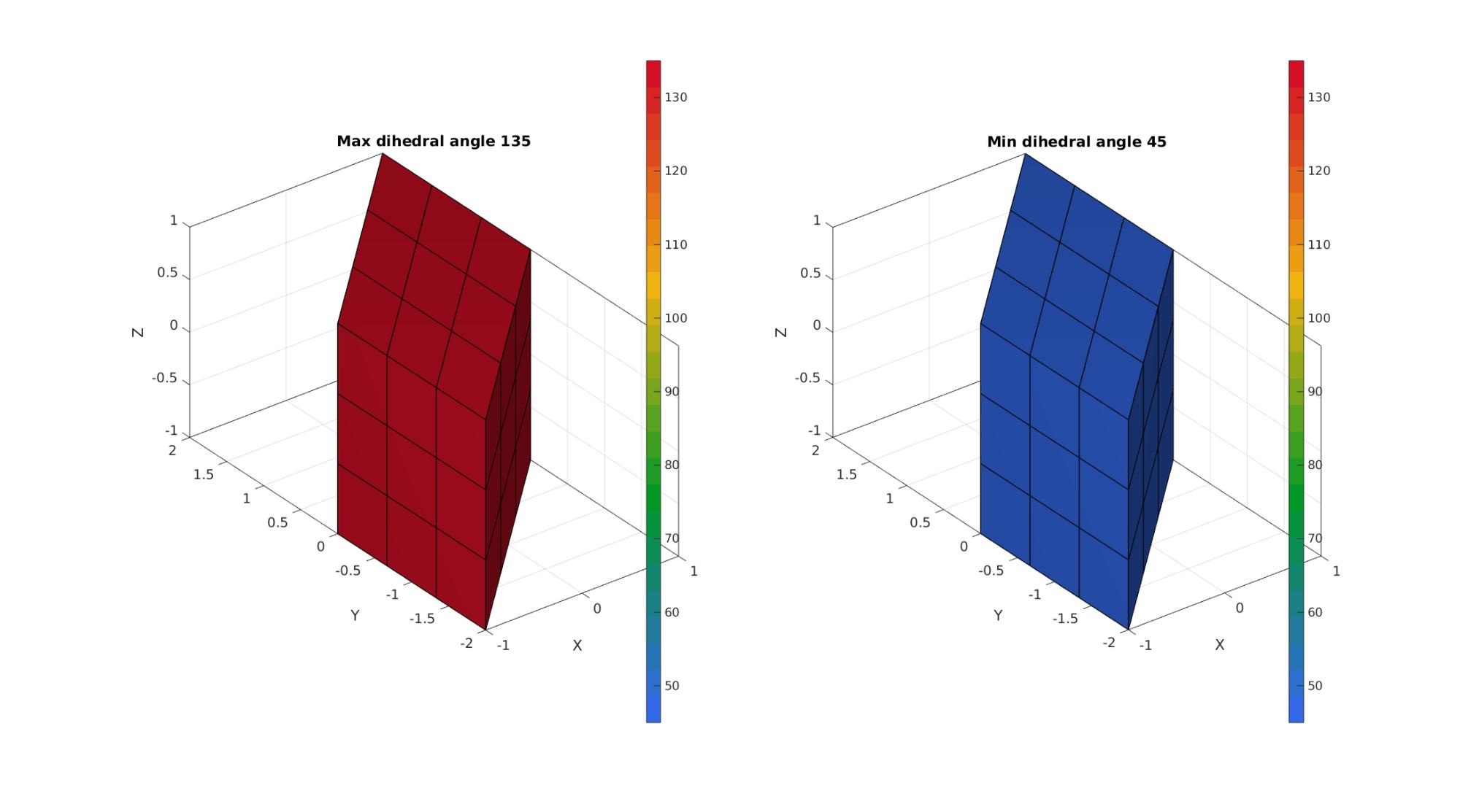# dihedralAngles

Below is a demonstration of the features of the dihedralAngles function

## Contents

```clear; close all; clc;
```

## Syntax

[A]=dihedralAngles(varargin);

UNDOCUMENTED

## Examples

Plot settings

```fontSize=20;
faceAlpha1=0.8;
```

## CREATING A MESHED BOX

```boxDim=2*ones(1,3);
boxEl=3*ones(1,3);
[meshStruct]=hexMeshBox(boxDim,boxEl);
E=meshStruct.E;
V=meshStruct.V;
```
```d=eye(3,3);
d(1,2)=1;

V=V*d;
```
```[A,EE,AE]=dihedralAngles(E,V,'hex8');
A=180*(A./pi);
AE=180*(AE./pi);

A_max=max(A,[],2);
A_min=min(A,[],2);

[F,A_max_F]=element2patch(E,A_max);
[~,A_min_F]=element2patch(E,A_min);
```
```cFigure;
subplot(1,2,1); hold on;
title(['Max dihedral angle ',num2str(max(A_max_F))])
gpatch(F,V,A_max_F,'k',1,1);
colormap(gca,gjet(25)); colorbar;
clim([min(A(:)) max(A(:))]);

subplot(1,2,2); hold on;
title(['Min dihedral angle ',num2str(min(A_min_F))])
gpatch(F,V,A_min_F,'k',1,1);
colormap(gca,gjet(25)); colorbar;
clim([min(A(:)) max(A(:))]);
gdrawnow;
```GIBBON www.gibboncode.org

Kevin Mattheus Moerman, [email protected]

GIBBON footer text

GIBBON: The Geometry and Image-based Bioengineering add-On. A toolbox for image segmentation, image-based modeling, meshing, and finite element analysis.

Copyright (C) 2006-2022 Kevin Mattheus Moerman and the GIBBON contributors

This program is free software: you can redistribute it and/or modify it under the terms of the GNU General Public License as published by the Free Software Foundation, either version 3 of the License, or (at your option) any later version.

This program is distributed in the hope that it will be useful, but WITHOUT ANY WARRANTY; without even the implied warranty of MERCHANTABILITY or FITNESS FOR A PARTICULAR PURPOSE. See the GNU General Public License for more details.

You should have received a copy of the GNU General Public License along with this program. If not, see http://www.gnu.org/licenses/.